Function Repository Resource:

FCGRImage

Produce a Frequency Chaos Game Representation image from a string of nucleotides

Contributed by: Daniel Lichtblau
 ResourceFunction["FCGRImage"][str] gives the Frequency Chaos Game Representation image of a DNA nucleotide sequence str comprised of the characters "A","T","G" and "C". ResourceFunction["FCGRImage"][str,k] gives the FCGR image at resolution 2k. ResourceFunction["FCGRImage"][str,k, bases] gives the FCGR image using a square with corners given by bases, beginning at the lower right and proceeding counterclockwise.

Details and Options

ResourceFunction["FCGRImage"][str] uses a default pixelation level of 7 and works with the square having "A" as the lower-right vertex, proceeding counterclockwise with "T", "G" and "C".
ResourceFunction["FCGRImage"][str,k] gives an image of dimension 2k×2k.
For ResourceFunction["FCGRImage"][str,k,bases], the third argument must be a permutation of the list {"A","C","G","T"}.
Any occurrence of the character "U" in str is converted to "T".
All characters in the converted input str other than {"A","C","G","T"} will be removed.
There is an “off-centered” asymmetry of the nucleotide positions from using grids that have side lengths of powers of 2. This will be illustrated in examples. It can be removed by enlarging the grids by one unit in each dimension.
By default we do not remove the asymmetry. The body of literature on the FCGR gives no indication of doing this. Perhaps more importantly, empirical evidence suggests that results are better when working with the slightly off-centered version.

Examples

Basic Examples

Create a “random” FCGR image using a pseudorandom string of nucleotides:

 In:=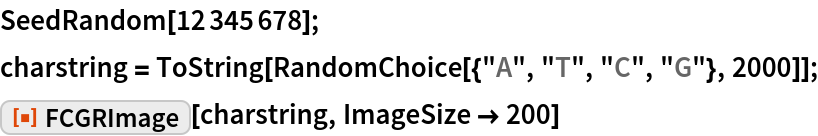Out=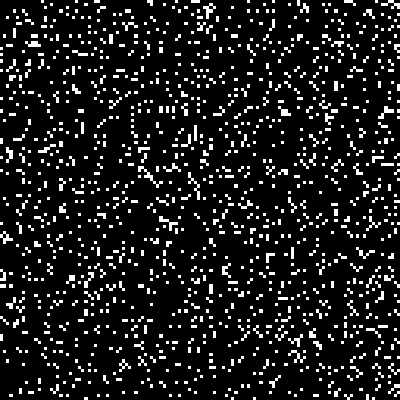Show simple images using sequences of the same nucleotide repeated three times:

 In:=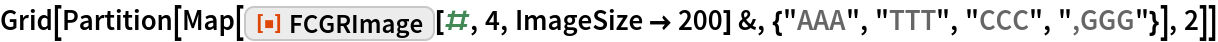Out=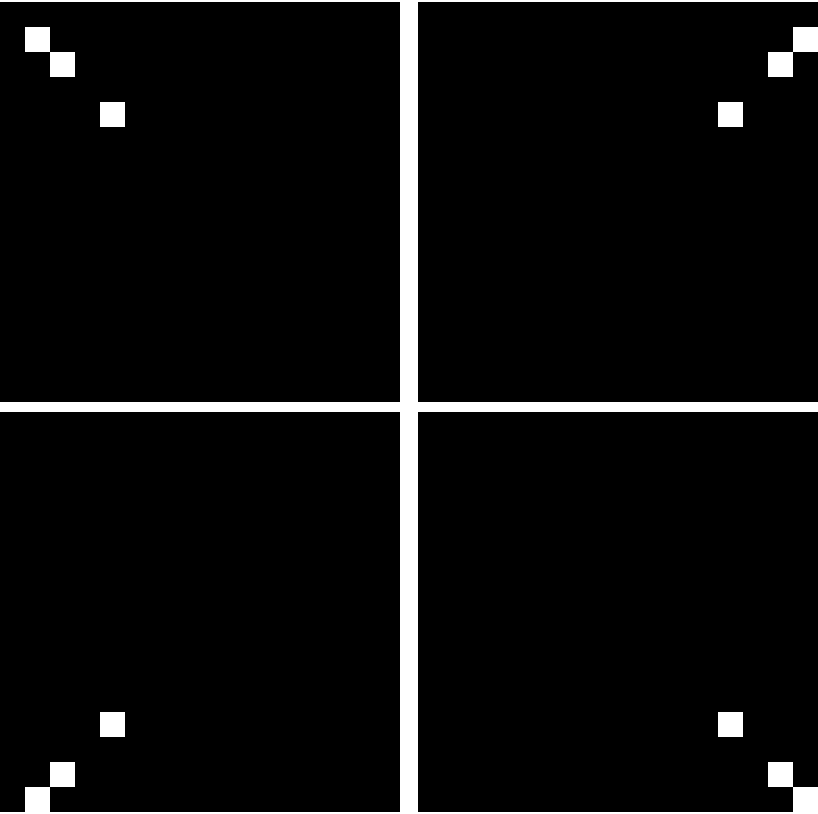The off-center asymmetry seen above is perhaps more apparent when we use sequences of length four:

 In:=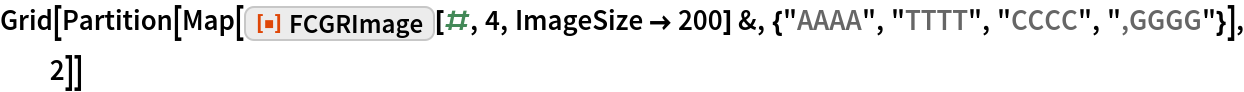Out=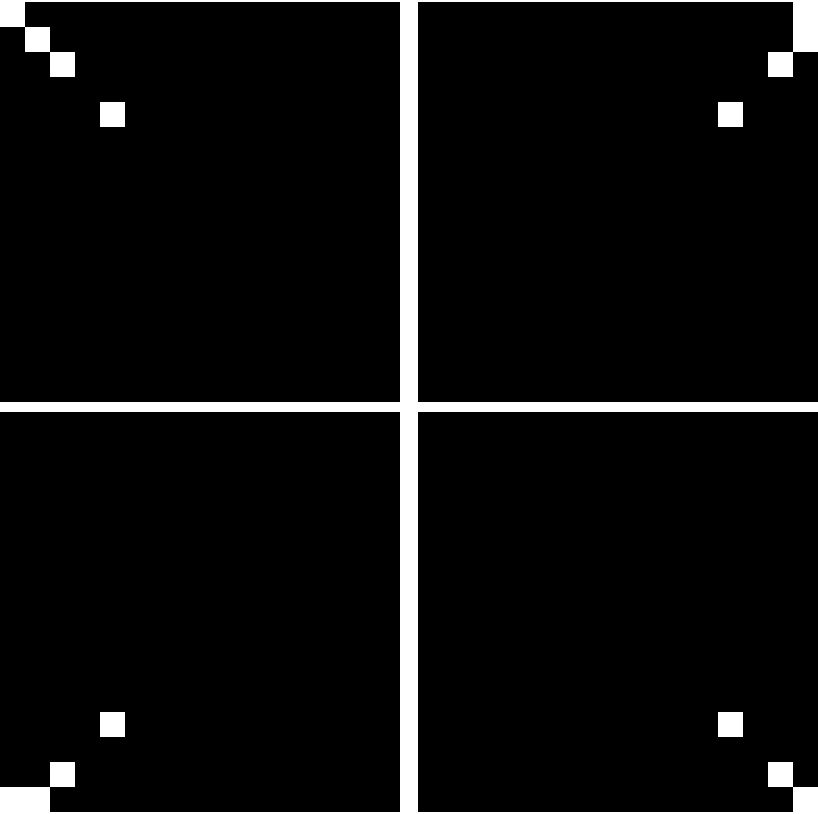The asymmetry can be removed using the option setting "Centered"True:

 In:=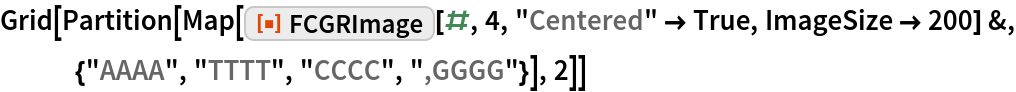Out=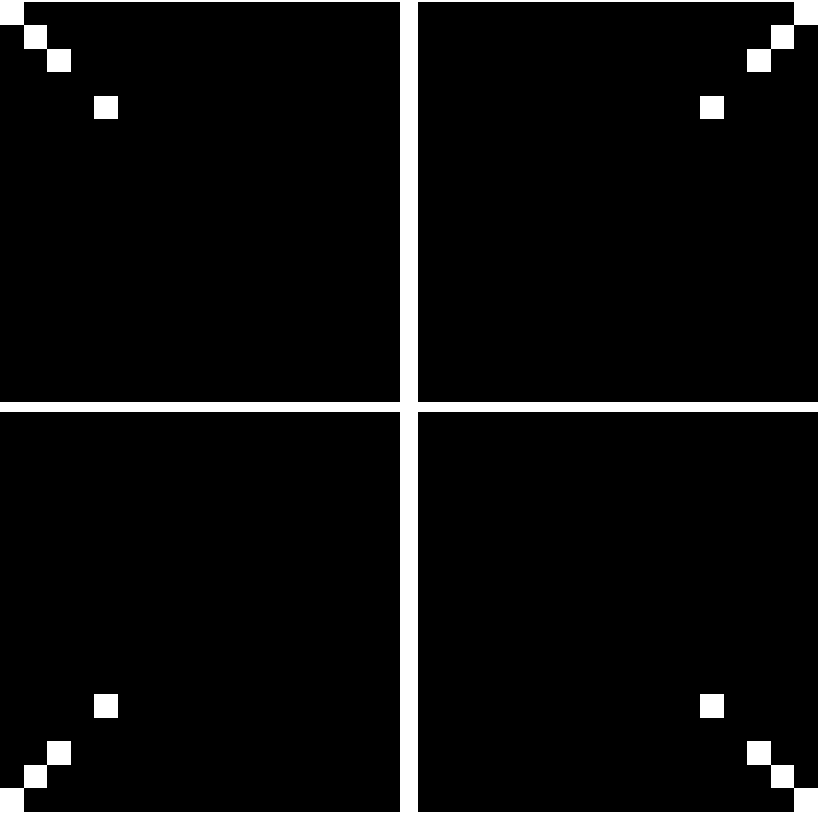Scope

Select a human gene from the Wolfram Language curated data:

 In:=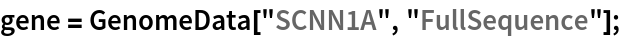Show the Chaos Game Representation image for this gene:

 In:=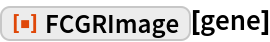Out=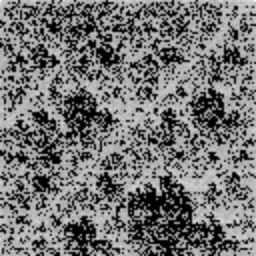Show this gene at higher levels of pixelation:

 In:=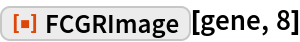Out=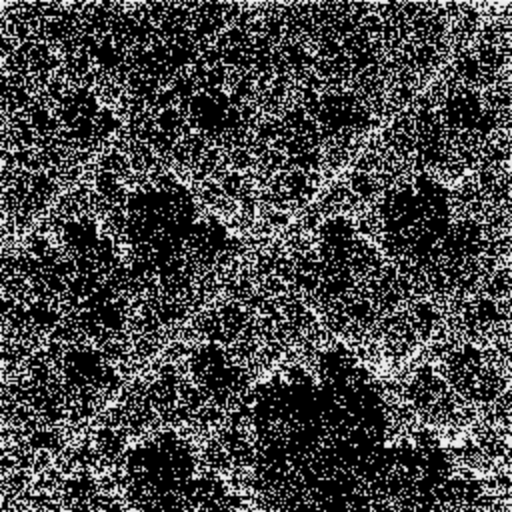One may observe a fractal nature to these, with similarities appearing as the resolution level is increased:

 In:=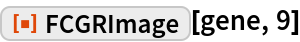Out=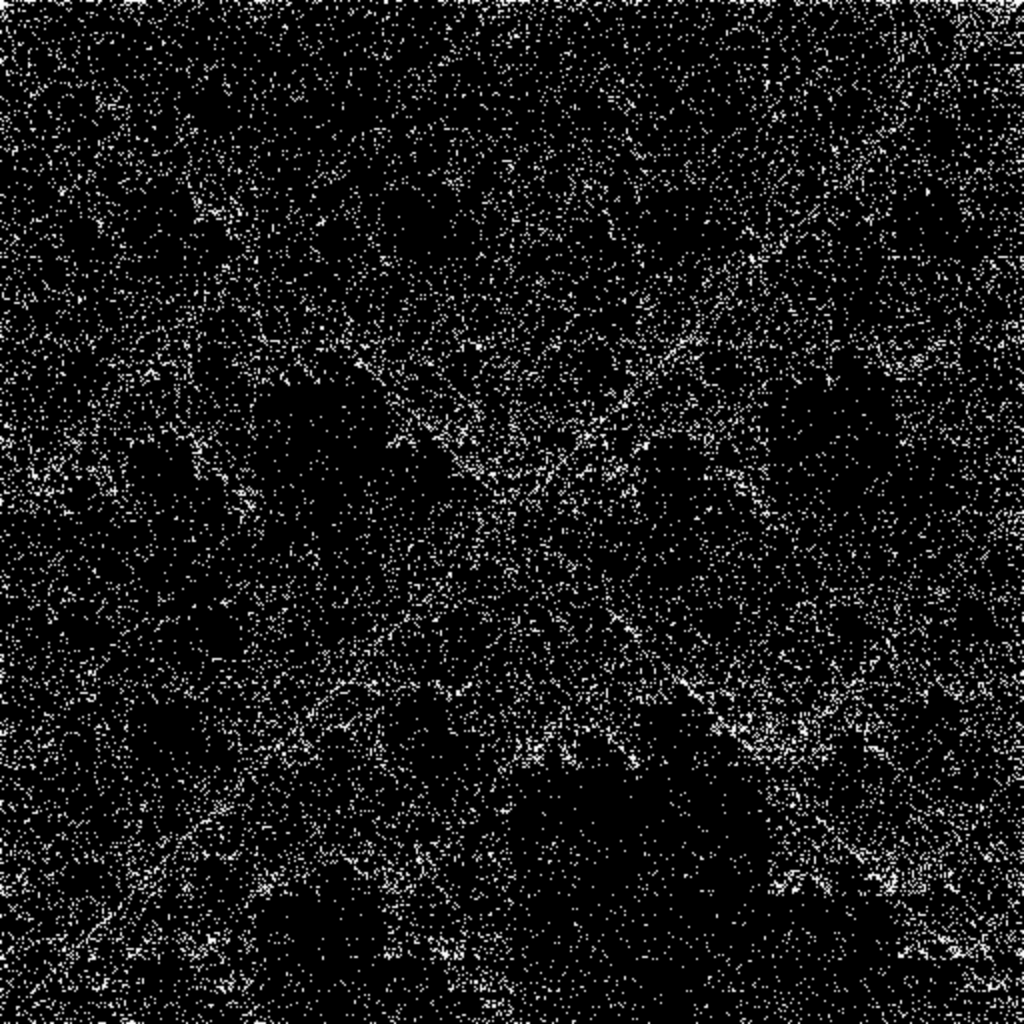Compute the image with respect to a square having the purines positioned at the bottom vertices and the pyrimidines at the top:

 In:=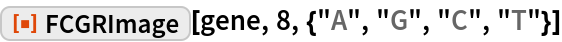Out=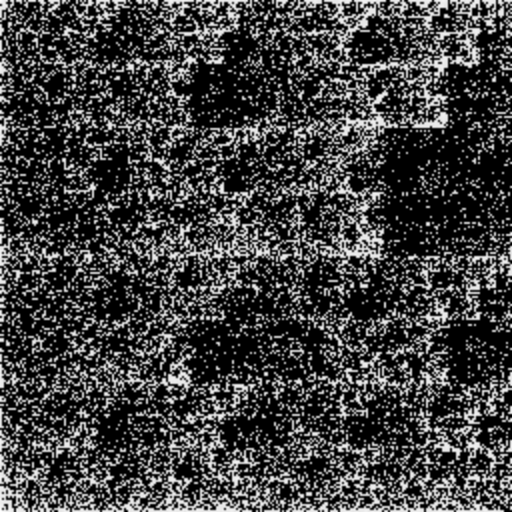Reverse the order of the purines from the default ordering:

 In:=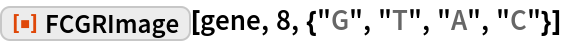Out=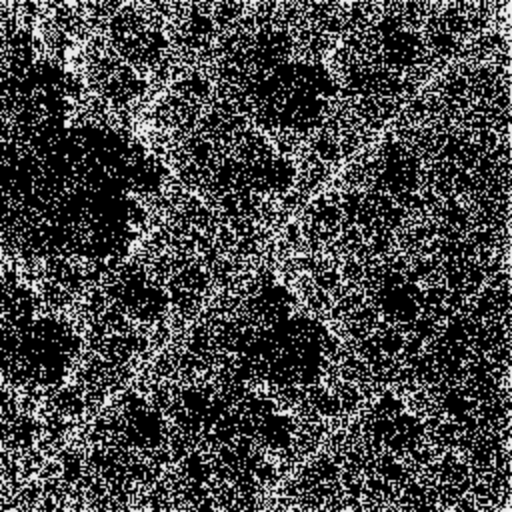Requirements

Wolfram Language 11.3 (March 2018) or above